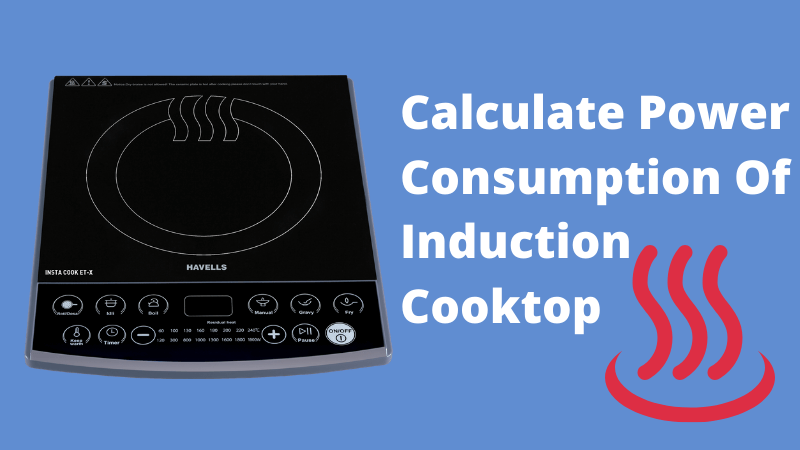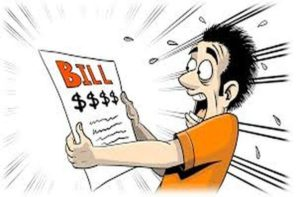## Calculate Power Consumption of an Induction Cooktop:More an more people are opting for induction cooktop or electric cooktop for cooking because of the hassle free cooking setup and less space occupancy. Power consumption of an induction cooktop or for that matter any appliance depends on its rated power and operational hours. On an average the rated power of an induction cooktop is somewhere between 1500 watts to 3000 watts, which is way higher than majority of the household appliances.

The higher the wattage, faster is the heating and less is the cooking time.

You can check the label on your induction cooktop to get the exact wattage or go to amazon and find your induction cooktop and check the wattage in description.

To use the induction cooktop power consumption calculator to calculate the power consumption of your electric cooktop and interpret the results properly you should understand the following terms.

If you already understand these terms feel free to skip the following section and go use the energy consumption calculator.

• What is Watt (W) – Watt is a unit of power. It means the rate at which electricity is consumed or produced by a device. For example a 50 watt TV consumes power at a rate of 50 watts per hour, it does not mean that the TV consumed 50 units of electricity, it means it will consume power at a rate of 50 watts every hour.
• What is Kilowatt (kW) – Kilowatt is also a unit of power. Kilowatt (KW) is used for large devices that consume more power. For example a 1.5 kW air conditioner, 6 kW elevator. There are bigger units like Megawatt (MW), Gigawatt (GW) to describe large power consumption or generation. For example 100 Megawatt (MW) coal power plant. (1 kW = 1000 W)
• What is a Kilowatt hour (kWh) – Units as described in electricity bill a.k.a kilowatt hour is the energy consumption of a device. For example a 50 watt Television (TV) running for 50 hours will consume 50 watt x 50 hours = 2500 watt hours = 2.5 kilowatt hours of electricity = 2.5 units of electricity. (1 kWh of electricity = 1 unit of electricity)
• Electricity Tariff – Electricity tariff is the amount the electricity provider charges you for one unit (kWh) of electricity. I live in Mumbai, India here the electricity tariff is Rs 12/kWh. If you don’t know how to calculate your tariff then just divide your total bill by monthly power consumption and you will get your electricity tariff.
• What is operational hours – Operational hours of a device refers to how many hours the device is working. If you run your television for 3 hours then operational hours of your television is 3 hours.

To calculate the power consumption of your induction cooktop just put the wattage of your induction cooktop, operational hours and electricity tariff of your locality and you will get the units of electricity consumed by your induction cooktop and the impact it has on your electricity bill.

If you don’t know the rated power of your induction cooktop then take it 2000 watts.

## Induction Cooktop Power Consumption calculator:

### Check out related blogs:## TV Power Consumption in A Day?

If you are wondering how much power does my TV...## Why My Electricity Bill Is So High And How To Rectify It.

You get a message from your electricity provider that your...